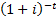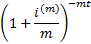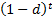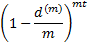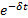/

### Annualisation Conventions: 2. ‘Interest’ rates vs ‘discount’ rates

Next section

In some circumstances, yields are not quoted in the form of interest rates but in the form of discount rates. Usually, ‘discount rate’ is used synonymously with ‘interest rate’, as a tool for calculating present values of future cash flows. However, in older literature the two terms may be distinguished, with discount rates being the way in which interest rates are traditionally quoted on discount bills. In such usage ‘discount rates’ would be expressed as fractions or percentages of the final terminal amount, rather than as fractions or percentages of the initial investment. So, if we invest 90 for one year and the terminal amount is 100 then the discount rate (with annual compounding), using this terminology, is 10%. More generally, if an amount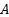is invested foryears at a discount rate, using this terminology, of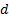that is compounded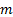times per annum then the terminal value of an investment is: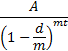Again we may consider the limit, as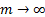. We find that the continuously compounded discount rate, using this terminology, is the same as the continuously compounded interest rate, since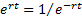.

In actuarial texts, the ‘effective’ interest and discount rates (i.e. annually compounded) are sometimes referred to byandrespectively and the ‘nominal’ interest and discount rates (compoundedtimes per annum) are sometimes referred to by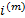and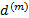respectively (in older texts,is sometimes instead referred to by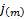). The ‘force’ of interest and the ‘force’ of discount (i.e. the continuously compounded rates) are the same and are sometimes referred to by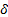. We then have the following relationships.

 Effective interest rateNominal rate of interestEffective rate of discountNominal rate of discountForce of interest or discountValue of 1 after time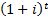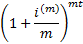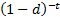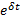Present value of 1 due after time